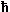# Phase and Group VelocityThe velocity v of the electron in the free electron gas model is the velocity with which the phase of the wave moves, it is called phase velocity vP.As long as a single plane wave symbolizes all that is to a particle, the phase velocity is automatically also the particle velocity v.This is no longer true, however, for particles that are described as a superposition of (plane or other) waves with all kinds of wave vectors - and that means most real particles.While each individual wave of the set that now describes the particle still travels with its individual phase velocity viP, the maximum of the total amplitude of all waves - signifying the most likely place to find the particle - travels with a velocity that must be identified with the particle velocity v and that is called group velocity.That the group velocity may be totally different from the phase velocity is nicely demonstrated by the example of standing waves, obtained by just combining two plane waves with wave vectors k and k . These waves have the same magnitude of the phase velocity, just opposite signs.The result is a standing wave with maxima and minima that are fixed in space; we have group velocity vG = 0. We may not really know where the particle is (locating it makes no sense under these coditions) but it certainly isn't going anywhere!The group velocity for a particle still characterized by one wave vector k as. e.g. in a Bloch wave, is calculated by the simple formula
vG  =  1·   dE(k)
dki.e. it follows directly from the dispersion relation E = E(k).This distinction is essential to avoid confusion between the over-simplified quantum mechanical picture of the free electron gas, and the treatment of electrons (and holes) as particles later on.While the electron in the free electron gas would move through the lattice with a rather large velocity because group and phase velocity are identical, the "real" electrons are rather sluggish.This is of course a direct consequence of the fact that only electrons and holes near the band edges are important where the group velocities are low because E(k) » constant.In other words: The electrons we are concerned with resemble much more standing waves than fast moving plane waves. Or, in yet other words: They spent a lot of time hanging around the atoms before they move on to some other atom.2.1.1 Essentials of the Free Electron Gas2.3.1 Effective Masses2.2.3 Life Time and Diffusion Length2.3.3 Shockley-Read-Hall RecombinationGain Coefficient

© H. Föll (Semiconductors - Script)